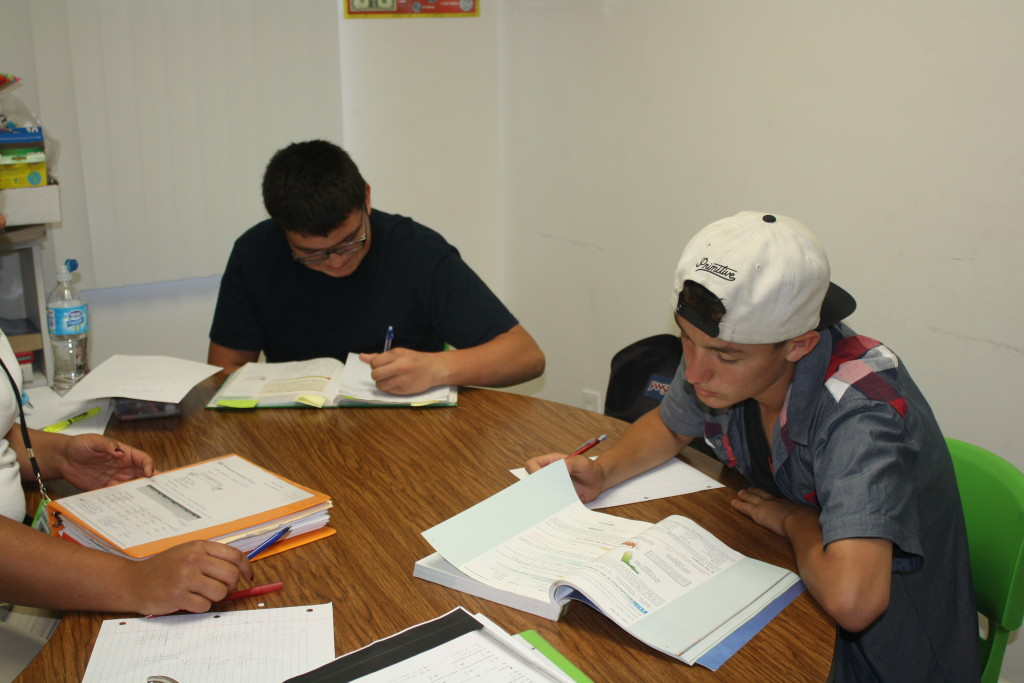# Calculus

###Tutoring 4 Less provides calculus tutoring to help your student succeed not only academically, but in the field they will pursue afterwards. Calculus is the branch of mathematics that studies rates of change. It is not only the foundation for all math, science, and technology fields, but is also a gateway to all the other advanced courses in mathematics. Calculus requires not only success in previous levels of math, all the way back to elementary school and middle school, but also a mastery of the major concepts introduced in each course. It specifically draws upon the skills mastered in Algebra I, II, and Trigonometry. Today calculus is used in every branch of the physical and natural sciences, computer sciences, statistics, engineering, economics, business, and medicine.

## Top Calculus Tutors Near Me in Los Angeles, CA

High school math doesn’t get any more challenging than calculus. It’s one of the subject areas in which private tutoring is most highly sought after, and conversely, at its scarcest. Many qualified math tutors prefer to focus on algebra, geometry, and other subject areas. However, Tutoring 4 Less offers some of the top calculus tutors in Los Angeles. Our tutoring staff have tutored students for years, working with a variety of learning styles and educational needs to guide students towards comprehension.

## Find An Online Tutor For Calculus

Since calculus is a required math subject for many majors and career fields, it has the reputation of being hard, or even impossible. Especially with online learning, students may lose motivation and give up calculus. Never fear – our online calculus tutors can help your student master calculus and gain confidence. Tutoring 4 Less offers affordable online tutoring for all math subjects, including calculus, with proven results. The tutors at Tutoring 4 Less are more than just great educators, they are positive mentors and influential role models. Our tutors consist of college students, college graduates and career educators that provide students with a positive outlook on education. We have adapted our proven calculus tutoring program to an effective online format, and our tutors are ready to help your child succeed in calculus and beyond.## What Does Calculus Tutoring Cover?

Calculus is divided into two major branches of study. One branch is Differential Calculus. In this course problems concerning rates of change, slopes and curves are addressed.The second branch of Calculus focuses on the calculation and accumulation of quantities and areas. They are related through the application of the Fundamental Theorem of Calculus.

Both branches make use of the fundamental notions of convergence of infinite sequences and infinite series to a well-defined limit.

Examples of typical differential calculus problems include:

• Finding the acceleration and velocity of a free-falling body at a particular moment
• Finding the optimal number of units a company should produce to maximize its profit.

Examples of integral calculus problems include:

• Finding areas and volumes of 3-Dimensional Shapes or Curves
• Finding the amount of water pumped by a pump with a set power input but varying conditions of pumping losses and pressure
• Finding the amount of parking lot plowed by a snowplow of given power with varying rates of snowfall

Tutoring 4 Less focuses on reinforcing a student’s understanding of the following major concepts needed in calculus:

• Real Numbers, Exponents, and Radicals
• Factoring
• Basics of Major Properties like Commutative, Associative, Distributive, Identitive and Density.
• Application of Major formulas like Distance, Area, Midpoint, Quadratic and Volumes.
• Application of Trigonometric functions and their Laws
• Understanding of Conic graphs and equations
• Slopes
• Matrices
• Vector and Polar Conversion Equations
• Sequences, Series and Probability

These concepts in calculus must be fully understood in order for our students to become successful. At Tutoring 4 Less, we go beyond learning the procedures and move toward having a conceptual understanding. In order to do this, we provide individual instruction in a small group or one-on-one setting to practice and work on a variety of problems. We are also happy to provide online tutoring sessions to students that cannot manage in-person tutorials for reasons of their own. Tutoring 4 Less makes sure each student fully understands how to arrive at their solutions and are able to prove their theories and processes.  Tutoring 4 Less also works on training our students to become active learners in every part of their education to become truly successful in all their Calculus courses.